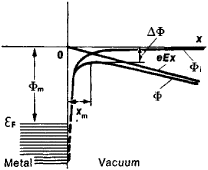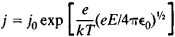# Schottky Effect

Also found in: Dictionary, Wikipedia.

## Schottky effect

The enhancement of the thermionic emission of a conductor resulting from an electric field at the conductor surface. Since the thermionic emission current is given by the Richardson formula, an increase in the current at a given temperature implies a reduction in the work function of the emitter. The reduction in work function can be calculated by considering the effect of a constant externally applied field on the potential energy of an electron near the conductor surface, and is found to be proportional to the square root of the field. See Thermionic emission, Work function (electronics)

A plot of the logarithm of the current versus the square root of the anode voltage should yield a straight line. An example is given in the illustration for tungsten; the deviation from the straight line for low anode voltages is due to space-charge effects. See Space charge

## Schottky Effect

a reduction in the work function of electrons emitted from solids that occurs under the influence of an applied electric field that accelerates the electrons. The Schottky effect is exhibited as an increase in saturation thermionic current, a decrease in surface ionization energy (seeION EMISSION), and a shift of the photoelectric threshold toward longer wavelengths λ. The effect occurs in electric fields E that are strong enough to neutralize the space charge at the surface of the emitter—that is, E ~ 10–100 volts per cm (V/cm)—and is substantial in fields up to E ~ 106 V/cm. At E > 107 V/cm, the tunneling of electrons through the potential barrier at the boundary of the solid begins to dominate; such tunneling results in field emission.Figure 1. The Schottky effect: (Φ) electron potential energy in the field of the image force, (eEx) electron potential energy in the applied electric field, (Φ) electron potential energy near the surface of the metal in the presence of the applied field, (Φm) work function of the metal, (ΔΦ) reduction in the work function under the influence of the applied field, (εF) Fermi level in the metal, (xm) distance from the height of the potential barrier to the surface of the metal; the shaded area indicates the filled electronic states in the metal

The classical theory of the Schottky effect for metals was developed by the German scientist W. Schottky in 1914. Owing to the high conductivity of metals, electric lines of force are perpendicular to the surface of a metal. Therefore, an electron, with a charge of – e, that is located at a distance of x > a, where a is the interatomic distance, from the surface interacts with the surface as if it induces its electric image—that is, a charge of + e —in the metal at a depth of x. The attractive force between the electron and its image is

(1) F = e2/16π∊0x2

where ∊0 is the permittivity of empty space. The potential of the force, or the image potential, Φi = –e/16π∊0x. The applied electric field reduces Φi by the quantity E × x (see Figure 1); a potential barrier with a maximum value at x = xm = (e/16π∊0E)½ appears at the metal-vacuum interface. At E ≤ 5 × 106 V/cm, xm ≥ 8 angstroms (Å). The reduction in the work function Φ caused by the applied field is ΔΦ = e(eE/4π∊0)½ For example, at E = 105 V/cm, ΔΦ = 0.12 electron volt and xm = 60 Å. As a result of the Schottky effect, the current j increases exponentially from j0 towhere k is the Boltzmann constant, and the photoelectric threshold frequency hω0 is shifted by the quantity

Δ(hω0) = e(eE/4π∊0)½

In the case where the emitting surface is nonuniform and covered with “spots” having different work functions, a “spot” electric field is produced on the surface. The “spot” field decelerates electrons emitted from cathode regions whose work functions are lower than those of adjacent regions. The applied electric field combines with the “spot” field and increases, suppressing the decelerating effect of the “spot” field. As a result, the emission current from a nonuniform emitter increases with increasing E more rapidly than in the case of a uniform emitter.

The effect of an applied electric field on the emission of electrons from semiconductors is more complicated. In semiconductors, the electric field penetrates to greater depths, ranging from hundreds to tens of thousands of atomic layers. Therefore, the charge induced by an emitted electron is located in a layer with a thickness of the order of the screening radius rs rather than at the surface. For x > rs, equation (1) is valid, but for fields E that are many times weaker than in the case of metals, that is, for E ~ 102–104 V/cm. In addition, an applied electric field that penetrates a semiconductor causes a redistribution of charges in the semiconductor, leading to an additional reduction in the work function. However, surface electronic states are usually present at the surface of a semiconductor. For a sufficiently high surface-state density (~1013 cm–2), the electrons in the surface states screen the applied field. In this case, the Schottky effect is the same as in the case of metals, provided the surface states are filled and emptied rapidly enough under the influence of an emitted electron’s field.

The Schottky effect is also observed when a current flows through a metal-semiconductor contact (seeSCHOTTKY BARRIER and SCHOTTKY BARRIER DIODE).

### REFERENCES

Schottky, W. Physikalische Zeitschrift, 1914, vol. 15, p. 872.
Dobretsov, L. N., and M. V. Gomoiunova. Emissionnaia elektronika. Moscow, 1966.
Nenakalivaemye katody. Moscow, 1974.

T. M. LIFSHITS

## Schottky effect

[′shät·kē i‚fekt]
(solid-state physics)
The enhancement of the thermionic emission of a conductor resulting from an electric field at the conductor surface.
Site: Follow: Share:
Open / Close